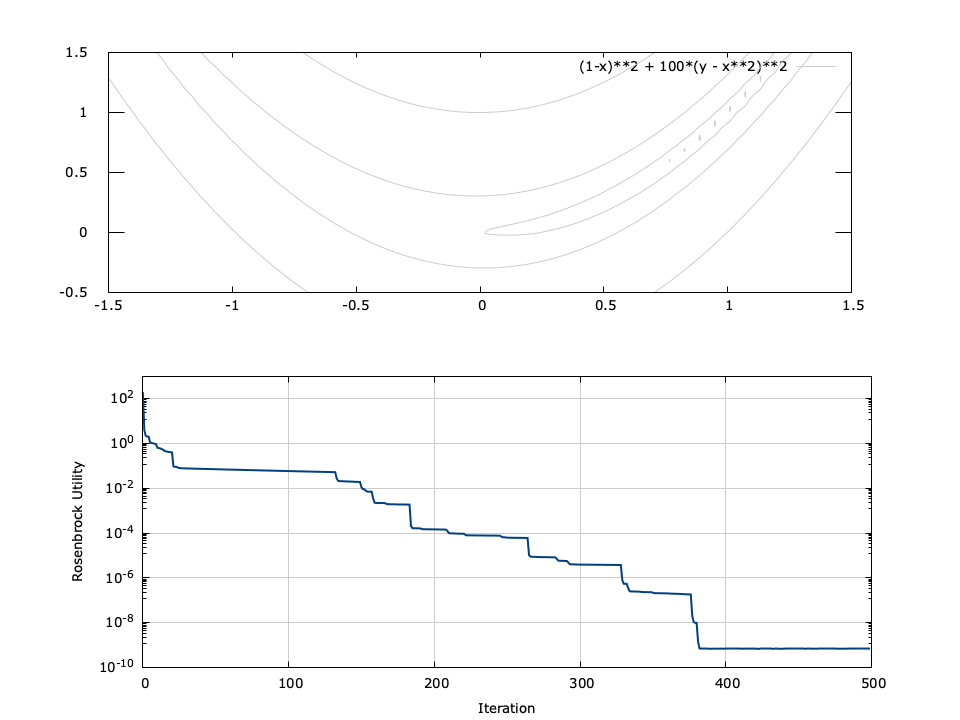This module implements a gradient or "steepest-descent" search. Given a function $$f$$ which operates on a vector$$\vec{x} = [x_0,x_1,\ldots,x_{N-1}]^T$$ of $$N$$ parameters, the gradient search method seeks to find the optimum $$\vec{x}$$ which minimizes $$f(\vec{x})$$ .

### Theory∞

The gradient search is an iterative method and adjusts $$\vec{x}$$ proportional to the negative of the gradient of $$f$$ evaluated at the current location. The vector $$\vec{x}$$ is adjusted by

$$\Delta \vec{x}[n+1] = -\gamma[n] \nabla f(\vec{x}[n])$$

where $$\gamma[n]$$ is the step size and$$\nabla f(\vec{x}[n])$$ is the gradient of $$f$$ at $$\vec{x}$$ , at the $$n^{th}$$ iteration. The gradient is a vector field which points to the greatest rate of increase, and is computed at $$\vec{x}$$ as

$$\nabla f(\vec{x}) = \left( \frac{\partial f}{\partial x_0}, \frac{\partial f}{\partial x_1}, \ldots, \frac{\partial f}{\partial x_{N-1}} \right)$$

In most non-linear optimization problems, $$\nabla f(\vec{x})$$ is not known, and must be approximated for each value of $$\vec{x}[n]$$ using the finite element method. The partial derivative of the $$k^{th}$$ component is estimated by computing the slope of $$f$$ when $$x_k$$ is increased by a small amount $$\Delta$$ while holding all other elements of $$\vec{x}$$ constant. This process is repeated for all elements in $$\vec{x}$$ to compute the gradient vector. Mathematically, the $$k^{th}$$ component of the gradient is approximated by

$$\frac{\partial f(\vec{x})}{\partial x_k} \approx \frac{f(x_0,\ldots,x_k+\Delta,\ldots,x_{N-1}) - f(\vec{x})}{\Delta}$$

Once $$\nabla f(\vec{x}[n])$$ is known, $$\Delta\vec{x}[n+1]$$ is computed and the optimizing vector is updated via

$$\vec{x}[n+1] = \vec{x}[n] + \Delta\vec{x}[n+1]$$

### Momentum constant∞

When $$f(\vec{x})$$ is flat (i.e. $$\nabla f(\vec{x})\approx \vec{0}$$ ), convergence will be slow. This effect can be mitigated by permitting the update vector equation to retain a small portion of the previous step vector. The updated vector at time $$n+1$$ is

$$\vec{x}[n+1] = \vec{x}[n] + \Delta\vec{x}[n+1] + \alpha\Delta\vec{x}[n]$$

where $$\Delta\vec{x} = \vec{0}$$ . The effective update at time $$n+1$$ is

$$\vec{x}[n+1] = \sum_{k=0}^{n+1}{\alpha^{k}\Delta\vec{x}[n+1-k]}$$

which is stable only for $$0 \le \alpha \lt 1$$ . For flat regions, the gradient vector $$\nabla f(\vec{x})$$ is approximately a constant $$\Delta\vec{x}$$ , and $$\vec{x}[n]$$ therefore becomes a geometric series converging to $$\Delta\vec{x}/(1-\alpha)$$ . This accelerates the algorithm across relatively flat regions of $$f$$ . The momentum constant additionally adds some stability for regions where the gradient method tends to oscillate, such as steep valleys in $$f$$ .

In liquid , the gradient is normalized to unity (orthonormal). That is $$\|\nabla f(\vec{x}[n])\|=1$$ . Furthermore, $$\gamma$$ is slightly reduced each epoch by a multiplier $$\mu$$

$$\gamma[n+1] = \mu \gamma[n]$$

This helps improve stability and convergence over regions where the algorithm might oscillate due to steep values of $$f$$ .

### Interface∞

Here is a summary of the parameters used in the gradient search algorithm and their default values:

• $$\Delta$$ : step size in computing the gradient (default $$10^{-6}$$ )
• $$\gamma$$ : step size in updating $$\vec{x}[n]$$ (default 0.002)
• $$\alpha$$ : momentum constant (default 0.1)
• $$\mu$$

Here is the basic interface to the gradsearch object:

• gradsearch_create(*userdata,*v,n,utility,min/max,*props) creates a gradient search object designed to optimize an $$n$$ -point vector $$\vec{v}$$ . The user-defined utility function and userdata structures define the search, as well as the min/max flag which can be either LIQUID_OPTIM_MINIMIZE or LIQUID_OPTIM_MAXIMIZE . Finally, the search is parametrized by the props structure; if set to NULL the defaults will be used. When run the gradsearch object will update the "optimal" value in the input vector $$\vec{v}$$ specified during create() .
• gradsearch_step(q) steps through a single iteration of the gradient search. The result is stored in the original input vector $$\vec{v}$$ specified during the create() method.
• gradsearch_execute(q,n,target_utility) runs multiple iterations of the search algorithm, stopping after either $$n$$ iterations or if the target utility is met.

Here is an example of how the gradient_search is used:

#include <liquid/liquid.h>

// user-defined utility callback function
float myutility(void * _userdata, float * _v, unsigned int _n)
{
float u = 0.0f;
unsigned int i;
for (i=0; i<_n; i++)
u += _v[i] * _v[i];
return u;
}

int main() {
unsigned int num_parameters = 8;    // search dimensionality
unsigned int num_iterations = 100;  // number of iterations to run
float target_utility = 0.01f;       // target utility

float v[num_parameters];            // optimum vector

// ... intialize v ...

v,
num_parameters,
&myutility,
LIQUID_OPTIM_MINIMIZE);

// execute batch searchFigure [fig-optim-gradsearch]. gradsearch performance for 2-parameter Rosenbrock function $$f(x,y) = (1-x)^2 + 100(y-x^2)^2$$ with a starting point of $$(x_0,y_0)=(-0.1,1.4)$$ . The minimum is located at $$(1,1)$$ .
[ref:fig-optim-gradsearch] depicts the performance of the gradient search for the Rosenbrock function, defined as$$f(x,y) = (1-x)^2 + 100(y-x^2)^2$$ for input parameters $$x$$ and $$y$$ . The Rosenbrock function has a minimum at $$(x,y)=(1,1)$$ ; however the minimum lies in a deep valley which can be difficult to navigate. From the figure it is apparent that finding the valley is trivial, but convergence to the minimum is slow.